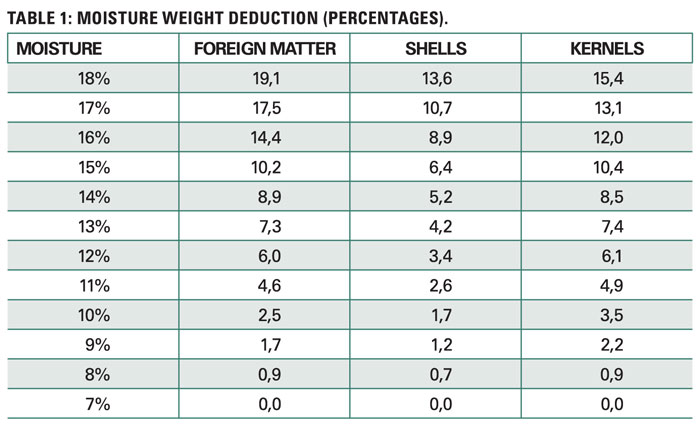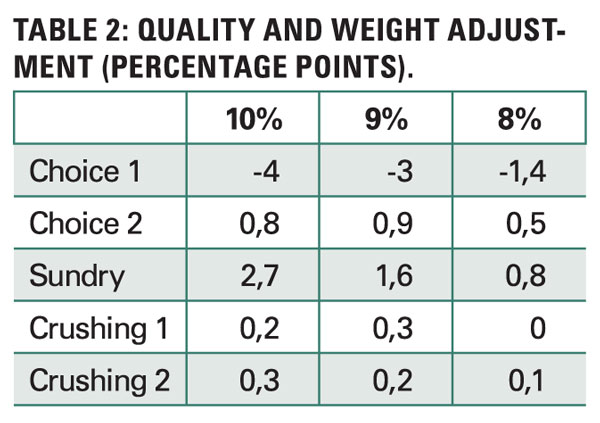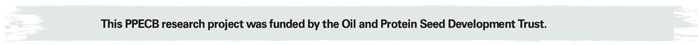• Search Icon

# Determine actual weight of wet pod groundnuts accurately

February 2018

##### NEELS WEGNER, product specialist: Gauteng, PPECB

Due to the increasing number of wet pod groundnuts received at the groundnut selection plants, it has more so become essential, to accurately determine the following:

• The weight loss per percentage point above 7% (kernel moisture) of foreign matter, groundnut shells and kernels, since these weight losses need to be deducted from the respective moisture percentage at which it was received.
• The quality and weight of groundnut kernels while it is still wet in pod (10% to 7% moisture) and then derive from that, what the groundnut quality and value will be once it has reached a 7% moisture level.

The groundnut industry is in need of a tabled scale of deductions to enable them to determine the accurate expected weight and value (at 7% kernel moisture) of wet pod groundnuts, at any moisture between 7% and 18%.How was the project conducted?
The analysing of the data took place in three stages.

Stage 1
Determination of the weight loss per percentage points of foreign matter and groundnut shells above 7%, since these weight losses need to be deducted from the respective moisture percentage at which it was received.

Data collection
Lot numbers were identified at different selection plants whereby the kernel moisture was above 7%, but preferably close to 18%.

A datasheet was then captured onto a lot number spreadsheet whereby a formula was used to calculate the weight at each moisture percentage as a percentage of the final weight at 7%.

A data spreadsheet was generated for each of the 28 samples that indicates the weights and percentage weight losses from 18% to 7% (at each percentage point above 7%), for all three grading aspects (foreign matter, shells and kernels).Data processing

• All the percentage calculations of each sample were compiled into Table 1.
• The average percentages were determined from all the results, with respect to all percentage points ranging from 18% down to 7%.
• From the above average percentages, a table was developed that indicate the suggested percentage deductions to be made from each percentage point above 7% for foreign matter, shells and groundnut kernels respectively.

Stage 2
Determine the quality and weight of groundnut kernels while it is still wet in pod (between 10% to 7% kernel moisture) and then derive from that which the groundnut quality/value will be once it reached a 7% moisture level.

Data collection
Lot numbers were identified at each selection plant whereby the kernel moisture was above 10%. All grading data was captured from a recording sheet onto a lot number spreadsheet whereby we insert formulas to calculate the percentages allocated to each grade at each of the moisture percentages from 10% - 7%.

For example: Choice 1 = 29,4%, Choice 2 = 38,5%, Sundry = 24,65%, Crushing 1 = 4,1% and Crushing 2 = 3,5%.

Data processing

• We compile all the percentage calculations of each sample (data spreadsheet) to a document – Table 2
• The average percentages of all the results from data spreadsheets were determined with respect to all percentage points ranging from 10% down to 7% for the different producer grades.
• From the above average percentages a table was developed that indicate the suggested percentage deductions to be made from each percentage point above 7% for Choice 1, Choice 2, Sundry, Crushing 1 and Crushing 2.

Stage 3
How to interpret and apply the results? From the above average percentages, two tables were developed that will indicate the suggested percentage deductions to be made from each research question’s perspective.

The average percentages of all the results have been determined, with respect to all percentage points ranging from 18% down to 7% on Table 1 and also all percentages from 10% - 7% on Table 2.

When kernels are wet and at an 18% moisture level (indicated in left column of Table 1), Table 1 can be used to indicate the percentage weight to be deducted from the original wet kernels, to determine the actual weight when it will be dry at a 7% moisture.

The deductions for foreign matter, shells or kernels can respectively be made from Table 1.

How to interpret the table: When ground­nuts are received at a kernel moisture of 18%:

• A deduction of 19,1% should be made from the foreign matter weight in order to determine the foreign matter weight at 7% moisture.
• A deduction of 13,6% should be made from the shells weight in order to de­termine the shells weight at 7% moisture.
• A deduction of 15,4% should be made from the kernel weight in order to determine the kernel weight at 7% moisture.

Example: When receiving 10 000 kg pods at a kernel moisture of 17% with a kernel content of 77%, it will be possible to determine the actual weight of kernels at a 7% kernel moisture level.

10 000 kg x 77% = 7 700 kg of kernels; minus 13,1% (from Table 2) = (10 000 x 77%) - 13,1% = 7 700 - 1 008,7 = 6 691,3 kg

Table 2 can be used to determine the quality and physical losses during the drying process from 10% to 7%.

Example: The producer grading of a consignment indicates the following percentages at a moisture level of 9%.
Choice 1 = 29%, Choice 2 = 39%, Sundry = 25%, Crushing 1 = 4%, Crushing 2 = 3%

The following calculations will be made in order to determine the percentages for each grade:
Choice 1 = 29% - 3% = 26%
Choice 2 = 39% + 0,9% = 39,9%
Sundry = 25% + 1,6% = 26,6%
Crushing 1 = 4% + 0,3% = 4,3%
Crushing 2 = 3% + 0,2% = 3,2%

Merit of the research
The merit and value of this research information as an addition to the Groundnut producer guidelines would be that it would be possible for groundnut graders to do a producer grading on a consignment where the moisture percentage is not yet regarded as dry.Publication: February 2018

Section: Focus on